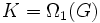# Full invariance does not satisfy image condition

This article gives the statement, and possibly proof, of a subgroup property (i.e., fully invariant subgroup) not satisfying a subgroup metaproperty (i.e., image condition).
View all subgroup metaproperty dissatisfactions | View all subgroup metaproperty satisfactions|Get help on looking up metaproperty (dis)satisfactions for subgroup properties
Get more facts about fully invariant subgroup|Get more facts about image condition|

## Statement

Suppose$G$ is a group,$K$ is a fully invariant subgroup of$G$, and$\varphi:G \to H$ is a surjective homomorphism. Then,$\varphi(K)$ need not be fully invariant in$H$.

## Proof

### Example of an Abelian group of prime-cube order

Suppose$G$ is the direct product of a cyclic group$A$ of order$p$ and a cyclic group of order$B$ of order$p^2$. Define:

•$K = \Omega_1(G)$ (see omega subgroups of a group of prime power order), i.e.,$K$ is the subgroup comprising all the elements:$\{ x \in G \mid px = 0 \}$.

•$\varphi$ is the quotient map by the normal subgroup$\mho^1(G)$ (see agemo subgroups of a group of prime power order), i.e.,$\varphi$ is the quotient map by the subgroup:$N := \{ y \in G \mid \exists x, px = y \}$.

• Observe that$K$ is fully invariant in$G$ (more generally, all omega subgroups are fully invariant). However,$\varphi(K)$ is a subgroup of order$p$ in$\varphi(G)$ which is elementary abelian of order$p^2$ -- hence$\varphi(K)$ is not fully invariant in$\varphi(G)$.

### Example of a non-abelian group of prime-cube order

Further information: Prime-cube order group:p2byp, Subgroup structure of prime-cube order group:p2byp

Let$p$ be an odd prime. Suppose$A$ is a cyclic group of order$p^2$ and$B$ is a cyclic group of order$p$, with$B$ acting on$A$ via multiplication by$p+1$. Then, the semidirect product of$A$ by$B$ is a non-Abelian group of order$p^3$. Call this group$P$. Define$\Omega_1(P)$ (see omega subgroups of a group of prime power order) as the subgroup generated by all elements of order$p$ in$P$. By the fact that Omega-1 of odd-order class two p-group has prime exponent,$\Omega_1(P)$ is a subgroup of prime exponent. This forces it to be a subgroup of order$p^2$ generated by the elements of$B$ and the multiples of$p$ in$A$. All the omega subgroups are fully characteristic, so$\Omega_1(P)$ is fully characteristic.

The center of$P$, namely$Z(P)$, simply comprises the multiples of$p$ in$A$. Thus, in the quotient map$P \to P/Z(P)$, the image of$\Omega_1(P)$ is cyclic of order$p$, while the whole group is elementary Abelian of order$p^2$. Thus:

•$\Omega_1(P)$ is fully characteristic in$P$.
• The image of$\Omega_1(P)$ in$P/Z(P)$ is not fully characteristic in$P/Z(P)$.